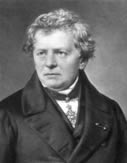# Ohm's Law and Poweraaaa George Ohm was a German physicist who studied the flow of charge through different resistors and was able to determine a mathematical relationship that we know today as Ohm's Law.

Discovery: Ohm discovered that, providing the temperature doesn't change too much, "The current I flowing through a resistor is directly proportional to the voltage V applied to the resistor"

Example - V is proportional to I

Ohm's Law can be expressed as a simple mathematical relationship,

Example - V = R I

This allows you to determine the resistance, R, of an electrical component by measuring the current that flows through the device and the potential difference (voltage) dropping over the device,

Example - R = V/I

The resistance of a material changes if the temperature of the resistor changes. When the temperature is increased - the resistance also increases, when the temperature is reduced - the resistance also reduces. This can be described simply as resistance is proportional to the temperature.

Power - the rate at which energy is transformed, i.e. the change in energy per second. When we think about electricity, we can see where the electrical power comes from by applying first principles, i.e. by thinking about it. A current is the number of coulombs per second flowing through a circuit and the voltage is a measure of the energy per coulomb. By multiplying the voltage by the current we have, coulombs/second x joules/coulomb.

By solving this you can see that the coulombs cancel out and we are left with joules/seconds.

This is the energy per second which we call the POWER and we can see that the power for an electric circuit can be calculated simply by multiplying the supply voltage by the current running into the circuit.

Example -  Power = voltage x current, or P = V I